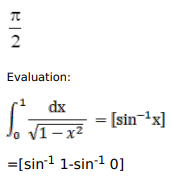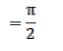# Solve this followingQuestion:

Evaluate:

$\int_{0}^{1} \frac{d x}{\sqrt{1-x^{2}}}$

Solution: